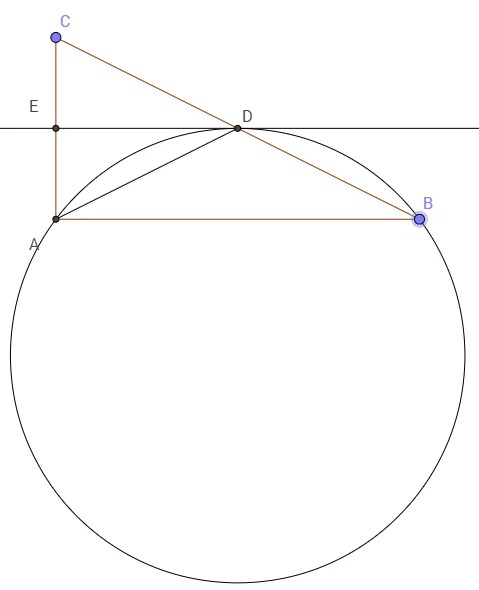Categories

# Orthogonality

Let ABC be a triangle and D be the midpoint of BC. Suppose the angle bisector of $$\angle ADC$$ is tangent to the circumcircle of triangle ABD at D. Prove that $$\angle A = 90^o$$ .

Discussion:Since DE is tangent to the circle at D, by tangent-chord theorem, we conclude:

$$\angle EDA =\angle ABD$$ (angle made by tangent with a chord is equal to the angle subtended by the chord in the alternate segment)…. (i)

But $$\angle EDA = \angle EDC$$ since DE is the angle bisector of $$\angle ADC$$. … (ii)

Hence combining (i) and (ii) we have $$\angle ABD = \angle EDC$$ implying DE is parallel to AB (as corresponding angles are equal).

Then $$\angle EDA = \angle DAB$$ (alternate angles) … (iii)

Combining (i) and (iii) we conclude $$\angle DBA = \angle DAB$$.

This implies $$\Delta ADB$$ is isosceles. Hence DA = DB.

But DB = DC (as D is the midpoint of BC.

Hence DA = DB = DC implying D is a point equidistant from the vertices A, B, C. Hence D is the center of the circumcircle of triangle ABC and BC is the diameter.

There $$\angle CAB = 90^o$$ (angle in the semicircle).## By Ashani Dasgupta

Pursuing Ph.D. in Geometric Group Theory at University of Wisconsin, Milwaukee